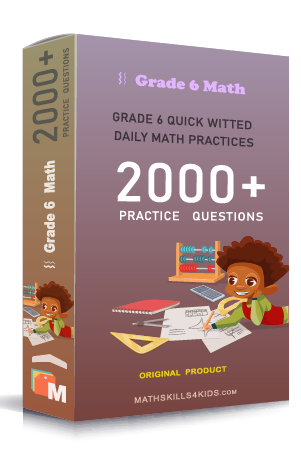# Grade 6 Exponents and square-roots worksheets with solutions

Subject
Math

Resource Type
Worksheets, Printables, Homeschool

Standards
CCSS 6.EE.A.1

Format
PDF (18.4 MB | 26 pages → Exercises + Solutions)

• ### -15% OFF Over \$100

Promo Code: TOPLA

After Purchase

\$3.15

This package allows you to practice the following skills

Writing multiplication expressions using exponents

Evaluating exponents

Writing powers of 10 with exponents

Finding the missing exponent or base

Evaluating exponents with decimal bases

Evaluating exponents with fractional bases

Understanding negative exponents

Evaluating negative exponents

Solving half-life word problems

Solving population doubling word problems

Finding square-roots of perfect squares

Estimating square-roots

This product is a part of the Mega Pack
* Grade 6 quick-witted daily math practices *